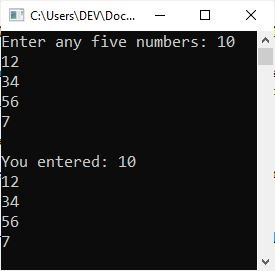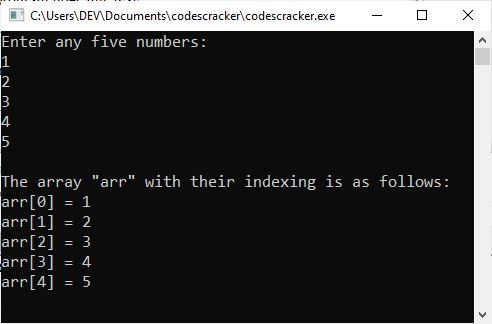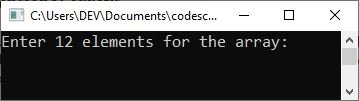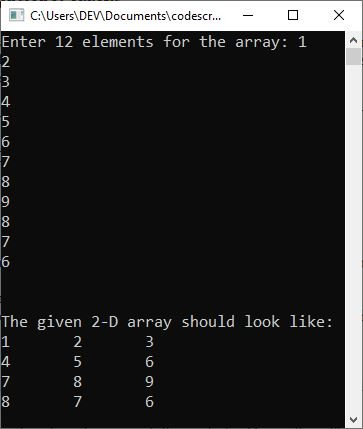# C++ Arrays with Examples

In this post, we are going to talk about "arrays in C++" and provide some examples. Therefore, let's not waste any more time and get right down to defining it shall we?

## What are arrays in C++

Arrays can be thought of as a user-defined type, but in order to define them in a C++ program, we have to use pre-defined data types like "int," "float," "char," etc.

In simple words if I define, then an array is a collection of values that can be stored in a single variable, but all the values must be of the same type. For example:

`int x;`

the above statement, declares an array named "x" of the type "int" that can store up to 10 numbers of the "int" type. Therefore, we can say that when we need to store multiple values in a single variable, we use array.

## In C++, declare an array

The general form of an array declaration in C++ is as follows:

`dataType arrayName[numberOfElement];`

where "dataType" refers to the type of element that should be stored in the array named "arrayName," and "numberOfElement" refers to the count. As an example:

`char a;`

declares a character-array with a size of 10, indicating that the array "a" can hold up to 10 characters.

## How to initialize arrays in C++

In C++, the general form for initializing arrays is as follows:

`type name[size] = {values};`

The "type" denotes the "data type," the "name" denotes the "name of the array," the "size" denotes the number of elements that the array can hold, and the "values" denotes the "comma-separated list of values." As an example:

`int arr = {97, 69, 18, 46, 83};`

Note: The number of values between the braces cannot be greater than the array size (here 5).

If you omit the array size, then the array becomes big enough to hold the initialization. Therefore, if you write:

`int arr[] = {97, 69, 18, 46, 83};`

The above statement created exactly the same array as the previous one. So, you can also initialize more or less than 5 values like this:

`int arr[] = {10, 12, 23};`

or

`int arr[] = {12, 23, 34, 35, 45, 33, 10, 2, 54};`

### Assign values to an array at a specific position in C++

Here is the general form to assign a value to a specific position in an array:

`array_name[index_number] = value;`

Here is an example of assigning a value of 20 to the fifth index of the array named "arr":

`arr = 20;`

Note: Since index always starts at 0, index number 4 corresponds to the 5th element in the array.

## How to access an array element in C++

By indexing the array name, an element can be accessed. This is accomplished by following the array name with the index number of the element enclosed in square brackets. In C++, the following is the general form for accessing an array element:

`type variable_name = array_name[index_number];`

Here's an example of how to get to the values in the array "arr" at index 4:

`int num = arr;`

So, if the value at arr is 20, the variable "num" will be initialized with 20.

## C++ arrays example program

Now is the time to look at a basic example of arrays in C++ to help clarify the concepts discussed above. So I wrote a program that prompts the user to enter any five numbers and then prints the entered five numbers back on the output console.

```#include<iostream>
using namespace std;
int main()
{
int arr;
cout<<"Enter any five numbers: ";
for(int i=0; i<5; i++)
{
cin>>arr[i];
}
cout<<"\nYou entered: ";
for(int i=0; i<5;  i++)
{
cout<<arr[i]<<endl;
}
cout<<endl;
return 0;
}```

The following snapshot shows the initial output produced by the above C++ program:Now type the first number, say 10, and hit the ENTER key; then type the second number, say 12, and hit the ENTER key; and so on. Following is the sample output after providing the five numbers one by one.The dry run of the above program goes this way:

• Using the statement:
`int arr;`
An array named "arr" is declared that can hold up to 5 values of the "int" type. And using the following first "for" loop, the program receives 5 inputs from the user and stores them in the array "arr" in such a way that the first element will be stored in "arr," the second element will be stored in "arr," and so on.
• And using the next "for" loop, I just printed all the elements of the array one by one.

Now let me modify the above program to print all elements of the array along with their indexing.

```#include<iostream>
using namespace std;
int main()
{
int arr;

cout<<"Enter any five numbers:\n";
for(int i=0; i<5; i++)
cin>>arr[i];

cout<<"\nThe array \"arr\" with their indexing is as follows:\n";
for(int i=0; i<5;  i++)
cout<<"arr["<<i<<"] = "<<arr[i]<<endl;

cout<<endl;
return 0;
}```

The following snapshots show the sample run of the above program with user inputs 1, 2, 3, 4, and 5 as the five elements of the array:### Calculate Array Size in C++

When the upper bound (UB) and lower bound (LB) of an array are given, then its size can be calculated as follows:

`Array_Size = (UB - LB) + 1`

where UB denotes the upper bound and LB denotes the lower bound. For example, if an array has elements numbered as follows:

`-7, -6, -5, ....0, 1, 2, ....15`

then its UB is 15 and LB is -7, and the array size can be calculated like this:

```= 15 - (-7) + 1
= 15 + 7 + 1
= 23```

Note: In C++, the lower bound is always 0 and the upper bound is size-1. (size specifies the number of elements in the array.)

In C++, all arrays consist of contiguous memory locations. The lowest address corresponds to the first element, and the highest address to the last element. Arrays are a way to group a number of items into the largest possible unit. Arrays can have data items of simple types like int or float or even of user-defined types like structures and objects.

## Types of Arrays in C++

Following are the two types of C++ arrays:

The "one-dimensional" array is discussed in a separate (next) post, whereas the "multi-dimensional" is going to be covered in this post, right after this paragraph.

### C++ multi-dimensional arrays

A multi-dimensional array is defined as a 2-D (two-dimensional) or more than 2-D array. Each element in a 2-D array can be considered an array (1-D array); in a 3-D array, each element can be considered a 2-D array; and so on. A 2-D array can be thought of as a matrix. For example:

`int mat;`

The above statement declares a 2-D array of size "4x3," where "4" is the "row size" and "3" is the "column size." It means that the array "mat" is able to store 12 elements, 3 elements in each column, making a total of 4 rows. For example:

```#include<iostream>
using namespace std;
int main()
{
int mat, i, j;
cout<<"Enter 12 elements for the array: ";
for(i=0; i<4; i++)
{
for(j=0; j<3; j++)
{
cin>>mat[i][j];
}
}

cout<<"\n\nThe given 2-D array should look like:\n";
for(i=0; i<4; i++)
{
for(j=0; j<3; j++)
{
cout<<mat[i][j]<<"\t";
}
cout<<endl;
}

cout<<endl;
return 0;
}```

The following snapshot shows the initial output produced by the above program:Now type the first element, say 1, and hit the ENTER key. Then type the second element, say 2, and hit the ENTER key again. In this way, provide 12 inputs as 12 array elements and hit the ENTER key finally to produce the following output:The dry run of the storing of array elements in the above program should be:

• When the user starts entering the 12 elements, then
• The first element will be stored at "mat."
• The second element will be stored at "mat."
• The third element will be stored at "mat."
• The fourth element will be stored at "mat."
• The fifth element will be stored at "mat."
• and so on up until
• The twelfth element will be stored at "mat."

That is, there are four one-dimensional arrays with three elements each.

The following code fragment demonstrates how to declare two-dimensional arrays in C++ and initialize them:

`int arr = { {1, 2}, {3, 4}, {5, 6}, {7, 8}, {9, 10} };`

While the next piece of code shows how to declare and initialize a three-dimensional array in C++:

```int arr = {
{
{1, 2, 3, 4},
{5, 6, 7, 8},
{9, 10, 11, 12}
},
{
{12, 14, 15, 16},
{17, 18, 19, 20},
{21, 22, 23, 24}
}
};```

For example:

```#include<iostream>
using namespace std;
int main()
{
int arr = {
{
{1, 2, 3, 4},
{5, 6, 7, 8},
{9, 10, 11, 12}
},
{
{12, 14, 15, 16},
{17, 18, 19, 20},
{21, 22, 23, 24}
}
};

for(int i=0; i<2; i++)
{
for(int  j=0; j<3; j++)
{
for(int k=0; k<4; k++)
{
cout<<"arr["<<i<<"]["<<j<<"]["<<k<<"] = "<<arr[i][j][k]<<"\t";
}
cout<<endl;
}
cout<<"\n\n";
}

cout<<endl;
return 0;
}```

The output of this example program on the three-dimensional array should exactly be:

```arr = 1        arr = 2        arr = 3        arr = 4
arr = 5        arr = 6        arr = 7        arr = 8
arr = 9        arr = 10       arr = 11       arr = 12

arr = 12       arr = 14       arr = 15       arr = 16
arr = 17       arr = 18       arr = 19       arr = 20
arr = 21       arr = 22       arr = 23       arr = 24```

That is, the three-dimensional array named "arr" defined in the preceding example is made up of two two-dimensional arrays, each of which is made up of three one-dimensional arrays, each of which has four elements.

Similarly, depending on your needs, you can work with multi-dimensional arrays in your C++ program.

#### More Examples

C++ Quiz

« Previous Tutorial Next Tutorial »